HOME / Applications / Eddy Current Testing: 3D simulation of probe with different excitation frequencies and crack sizes in EMS

# Eddy Current Testing: 3D simulation of probe with different excitation frequencies and crack sizes in EMS

Used Tools:## Eddy Current Testing

Eddy-current testing (ECT) is a non-destructive testing (NDT) method based on the electromagnetic induction. Its main applications is surface inspection, whose goal is detection of surface and sub-surface flaws in the specimen.

A single-element ECT probe has a coil of conductive wire which is excited from an AC source. This coil produces an alternating magnetic field in the surrounding space. The coil’s field induces eddy currents in the nearby conductive material. The direction of the eddy current is such that its field opposes the flux created by the coil. Flaw in the material causes variation in the electrical conductivity and magnetic permeability which changes the eddy current and the impedance ‘seen’ by the coil.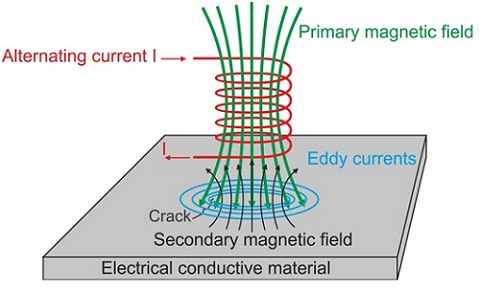Figure 1 - Eddy Current Testing principle

## Problem description

The example consists of conductor plate which is scanned by a coil. The crack on the plate is detected through a variation in the impedance of the coil.  Different crack sizes and excitation frequencies help to study the behaviour of the coil under several scenarios.EMS results are validated by experimental tests.

Below is the 3D model of a simulated probe. A parametric AC magnetic analysis is used to solve this example. The impedance of the coil is measured for different coil positions with -a step of 2 mm.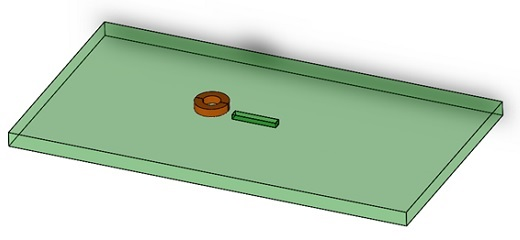Figure 2 - CAD model of simulated NDT example

Figure 3 shows the geometrical parameters of the model. The crack width and depth are 2mm and 1.3mm respectively. Two different crack lengths have been simulated- 12 mm and 8mm.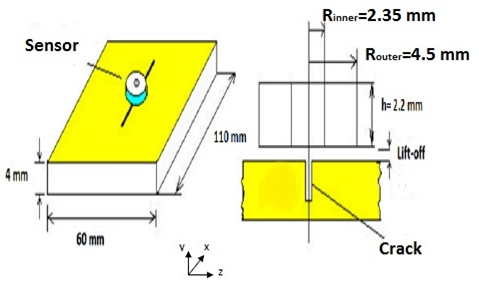Figure 3 - Geometrical parameters of the model

### Study

The EMS AC Magnetic module of is used to compute results such as magnetic flux density,eddy current density and loss density for a sinusoidal excitation. Other results given by EMS are total eddy current loss, Joule loss, impedance matrix,etc.For this example, a parametric analysis will provide impedance for each position of the coil.

These four steps should be followed to perform an AC Magnetic simulation inEMS:
1. Create a new AC Magnetic study
2. Apply suitable materials to the parts
3. Apply a suitable coil (either voltage or current driven) with the correct excitation.
4. Mesh and Run the simulation

### Materials

Both the coil and the plate are made of copper. Below are the properties of the copper and the air surrounding the plate and coil.

Table 1 - Copper properties

 Relative permeability Electrical conductivity S/m Copper 1 58.100e+6 Air 1 0

#### Coil

The sensor here is the coil. Table 2 contains the coil properties.

Table 2 - Coil properties

 Number of turns Current magnitude Wound Coil 170 20 mA

#### Mesh

Mesh is critical to all FEM simulation. The results accuracy and the solving time are strongly depending on the mesh size. According to FEA theory, the models with finer mesh (small element size) yield highly accurate results but will take longer computing time. On the other hand, those models with coarse mesh (large element size) may lead to less accurate results but faster computing time. Mesh can be generated automatically by EMS after estimating the global size of model. Also, EMS offers more meshing flexibility due to the possibility of controlling the mesh size on solid bodies and faces by the option of mesh control. In this example, a mesh control is applied on a small air surrounding the coil. The air in the crack is also finely meshed, with the maximum element dimension of 0.1mm.  Figure 4 shows the final mesh.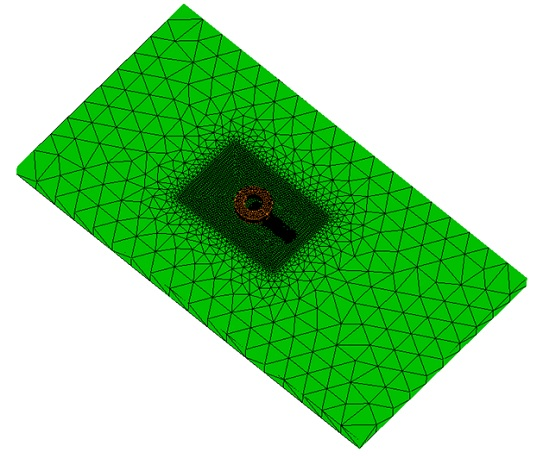Figure 4 - Meshed Model

### EMS results

After running the parameterized simulation with crack length of 12mm, coil lift-off of 0.13 mm and 50 kHz excitation frequency, the results are ready. A parametric analysis in EMS allows user to sweep between either geometrical or simulation parameters (current, number of turns, frequency, mesh size…). The results of all scenarios are given in a single study.

Figure 5 shows the current density distribution in the model without a crack. The eddy currents are concentered within the skin depth. Figure 6 shows the current density distribution when the coil is exactly above the center of crack.Figure 5 - Current density distribution in case of plate without crack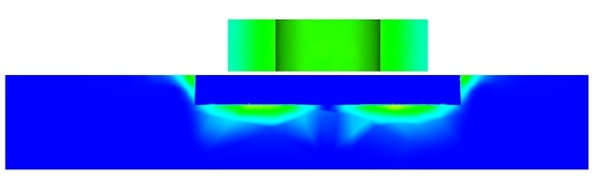Figure 6 - Current density distribution in case of plate with crack

The coil (probe) scans the plate in the direction of the crack length. Symmetry is used to limit the size of the problem, so only half of the coil positions are simulated – starting at the crack center towards its end. The impedance of coil is measured in each step. Figure 7 shows that the impedance is at maximum value in the center of crack. In Figure 8 a comparison is madebetween EMS and test results . Simulation results are very close to the measurements.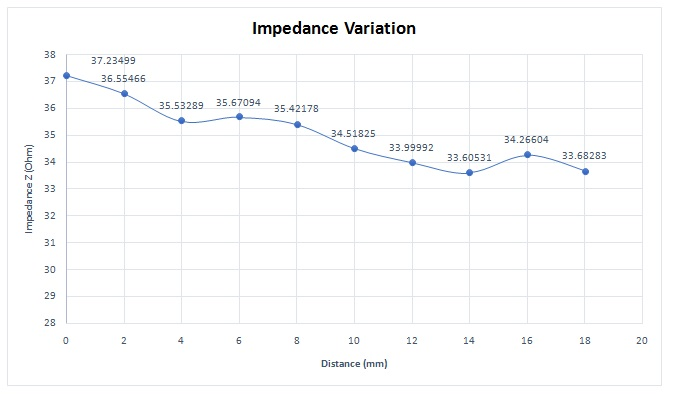Figure 7 - Impedance variation of coil sensor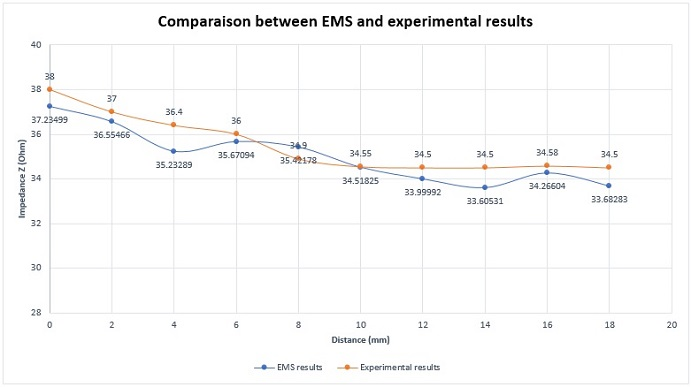Figure 8 - Comparison between EMS and experimental results for the impedance variation (50kHz and 12 mm crack length)

Figure 9 is representing the absolute change in the impedance of EMS and experimental tests. It can be calculated as shown in the formula below: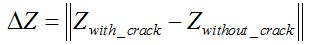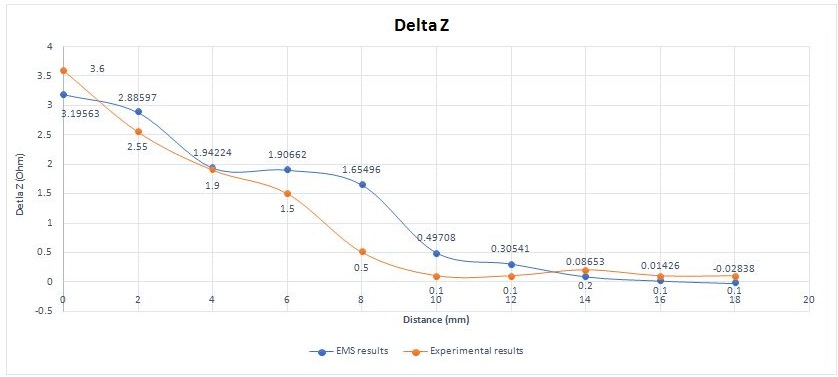Figure 9 - Comparison between EMS and experimental results for absoluteimpedancevariation (50kHz and 12 mm crack length)

### Relation between crack dimensions and captured signal

The crack size affects the impedance behaviour during the sensor scan. Figure 10 shows a comparison of the impedance variation for two different crack lengths.  The variation in the impedance with higher crack length is more important and could be detected easier.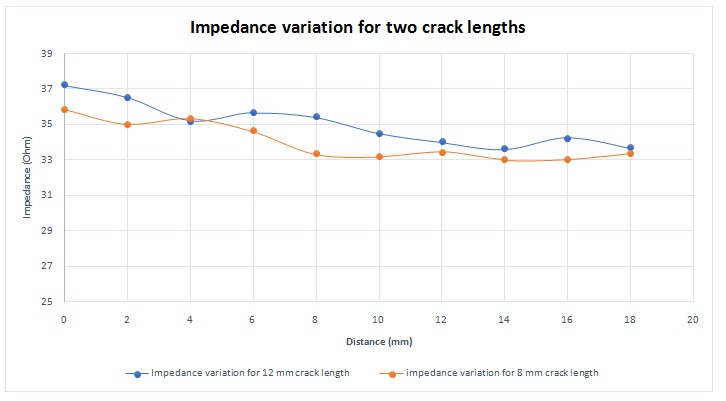Figure 10 - Impedance variation for different crack lengths

EMS simulation results match very well the experimental results as shown in figure 11.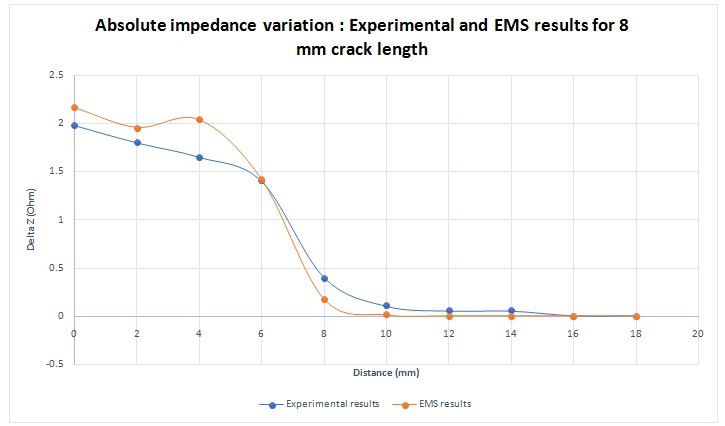Figure 11 - Absolute impedance variation for 8 mm crack length

### Relation between excitation frequency and captured signal

The coil can be excited with different frequencies. EMS and experimental results at Figure 12 demonstrate that crack detection is easierat higher frequencies.In fact, this deduction can be explained by the relationship between frequency and skin depth. The formula below shows on which parameters depends the skin depth: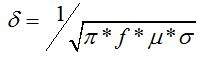Where f is the excitation signal frequency,is the material conductivity andis the material permeability.

The skin depth is inversely proportional to the frequency. So, a higher frequency gives a smaller skin depth. As a result, the generated eddy current will be closer to the plate surface and to the sensor also. A higher magnetic flux will be produced by this eddy current and affects more the probe.  Once again, EMS calculation and measured data are in very good agreement.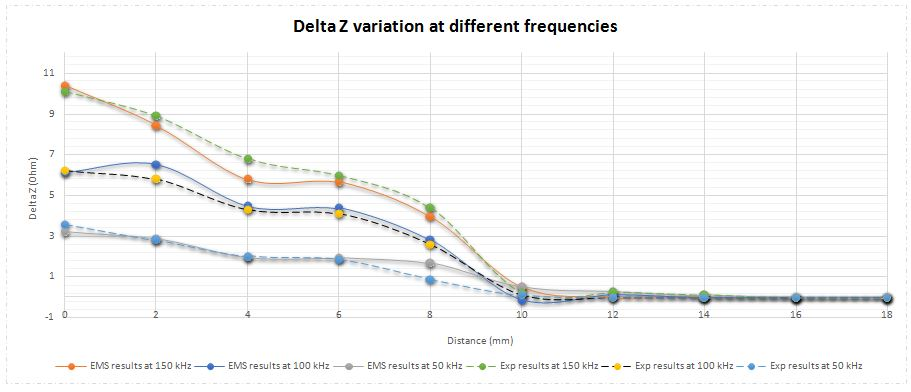Figure 12 - EMS and Experimental results of absolute impedance variation at different frequencies

### Relation between lift-off distance and captured signal

The distance between the coil sensor and the specimen surface is critical for accurate crack detection. As shown in figure 13, the variation of the absolute impedance is more pronounced smaller lift-off.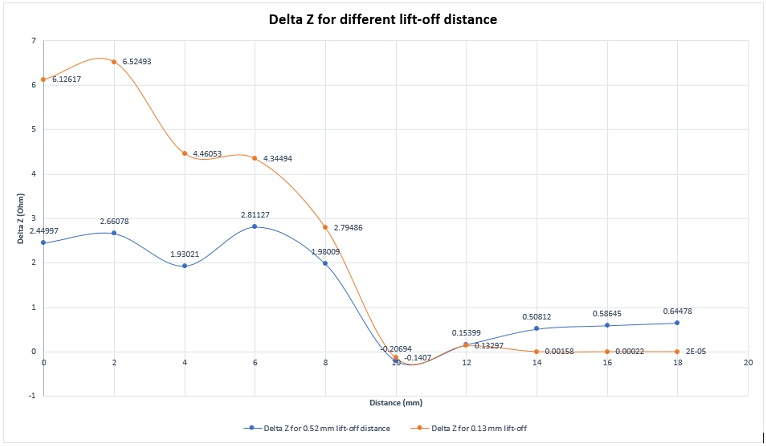Figure 13 - EMS results of absolute impedance variation for different lift-off distance

## Conclusion

EMS successfully simulates performance ofa variety of electromagnetic NDE systems. The presented example demonstrated in particular the capabilities of EMS for characterization of an eddy-current detector used for surface inspection. It easily takes into consideration all the important features of the sensor and the specimen. EMS results are confirmed by comparison with experimental data.

## References

:Hamel Meziane.2012. Etude et réalisation d ‘un dispositive de détection de défaut par méthodes électromagnétiques.

Upcoming Webinars